# FSC Math Full Book MCQs 2

NUST entry test 2023 NET contains most of the Mathematics MCQs. FSC Part 2 Math book MCQs. HSSC Math questions MCQs. Solved Chapterwise Math MCQs for first-year 12 class MCQs. Second-year and first-year Math textbooks chapter-wise MCQs with the answer are provided here. PakLearningSpot PLS MCQs Bank website for FREE Entry test preparations

0%
146
Created by Ali Durrani

NET Practice

Math Practice Test 2

1 / 100

The method of induction was given by Francesco who lived from:

2 / 100

Parallelogram law of vector addition to describe the combined action of two forces, was used
by

3 / 100

7. The equations involving redical expressions of the variable are called:

4 / 1005 / 1006 / 100

Product of cube roots of -1 is:

7 / 100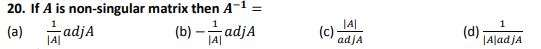8 / 100

In the cases of ellipse it is always true that:

9 / 100

The circle whose radius is zero is called:

10 / 100

The complex cube roots of unity are………………. each other.

11 / 100

To determine the area under the curve by the use of integration , the idea was given by

12 / 100

Inverse of any element of a group is:

13 / 100

12. General solution of every trigonometric equation consists of :

14 / 100

Equation of vertical line through (−?, ?) is

15 / 100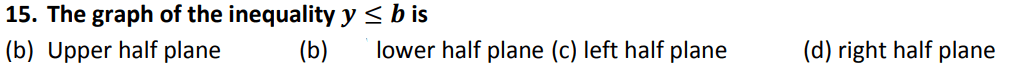16 / 100

If the cross product of two vectors is zero, then the vectors must be

17 / 100

𝒔𝒊𝒏(𝟐𝝅 − 𝜽) =

18 / 100

The equation 𝒚 = 𝒙𝟐 − 𝟐𝒙 + 𝒄 represents ( 𝒄 being a parameter )
(Error Comment below the anwser)

19 / 100

The angle subtended at the center of a circle by an arc whose length is equal to the radius of
the circle is called:

20 / 10021 / 100

If a graph express a function , then a vertical line must cut the graph at

22 / 100

If line ? intersects ? − ???? at a point (?, ?) , then the ? − ????????? of the line ? is:

23 / 100

Straight line angle is equal to

24 / 100

The equation in which variable occurs in exponent , called:

25 / 100

All lines through vertex and points on circle generate a

26 / 100

60th part of ?° is equal to

27 / 100

Which of the following is not a binary operation :

28 / 100

The symbols used for inequality are

29 / 100

The complex fourth roots of unity are ……. of each other.

30 / 100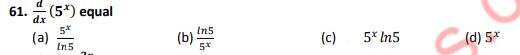31 / 100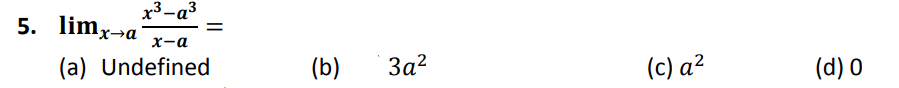32 / 100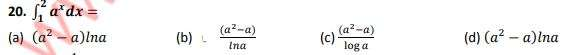33 / 100

Straight line angle is equal to

34 / 10035 / 100

All trigonometric functions are ……………….. functions.

36 / 100

The set of points which are equal distance from a fixed point is called:

37 / 100

Which one is related to circle

38 / 100

𝑹 − {𝟎} is a group 𝒘. 𝒓. 𝒕 the binary operation

39 / 100

The multiplicative identity of complex number is

40 / 100

Range of 𝒚 = 𝒄𝒐𝒔𝒙 is

41 / 100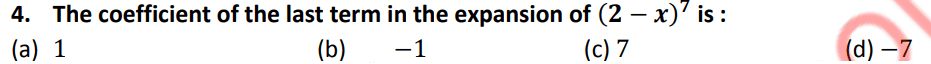42 / 100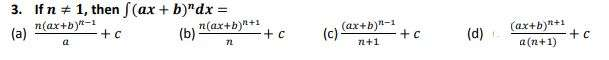43 / 100

If order of 𝑨 is 𝒎 × 𝒏 and order of B is 𝒏 × 𝒒 then order of AB is

44 / 10045 / 10046 / 100

The general term of a ?. ?., is :

47 / 10048 / 100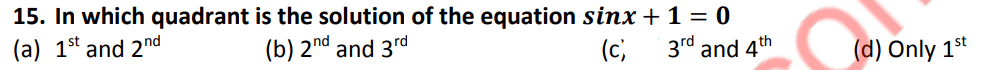49 / 100

(𝒙 + 𝟑)(𝒙 + 𝟒) = 𝒙^𝟐 + 𝟕𝒙 + 𝟏𝟐 is a/an:

50 / 10051 / 100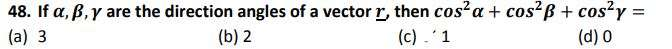52 / 100

If a matrix A has different number of rows and columns then A is called:

53 / 100

The high school math department needs to appoint a new chairperson, which will be based on seniority. Ms. West has less seniority than Mr. Temple, but more than Ms. Brody. Mr. Rhodes has more seniority than Ms. West, but less than Mr. Temple. Mr. Temple doesn’t want the job. Who will be the new math department chairperson?

PreMedPK MDCAT Mock 5
Logical Reasoning
Logical Problems
Passage
Elimination Tool:

54 / 10055 / 100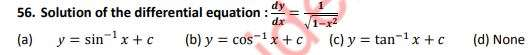56 / 100

If 𝑨 ⊆ 𝑩 then 𝒏(𝑨 ∩ 𝑩) =

57 / 100

The lines joining the mid-points of any two sides of a triangle is always _____to the third side.

58 / 100

The term “Function” was recognized by______ to describe the dependence of one quantity
to another.

59 / 100

Two rays with a common starting point form:

60 / 100

If all the sides of four sided polygon are equal but the four angles are not equal to ??° each
then it is a

61 / 100

Period of 𝒕𝒂𝒏𝜽 is

62 / 100

The set {𝟏, −𝟏} possesses closure property 𝒘. 𝒓. 𝒕

63 / 100

When we look an object above the horizontal ray, the angle formed is called angle of:

64 / 100

Squaring a number is a:

65 / 10066 / 100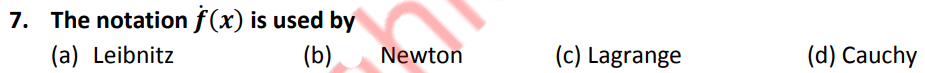67 / 100

The angle in semi-circle is equal to:

68 / 100

If any two vectors of scalar triple product are equal, then its value is equal to

69 / 100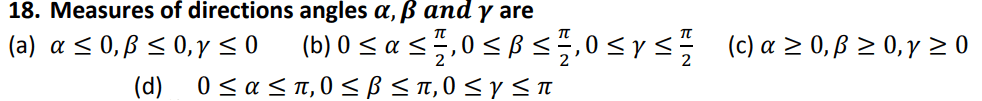70 / 100

Squaring a number is a:

71 / 100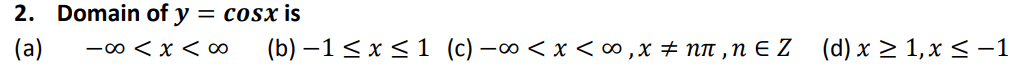72 / 100

The symbol which is used to denote negation of a proposition is

73 / 100

∫???+?? =

74 / 100

The graph of linear function is :

75 / 100

The number of diagonals in 10-sided figure is

76 / 100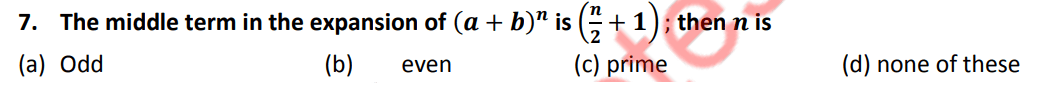77 / 100

The symbols used for inequality are

78 / 10079 / 10080 / 100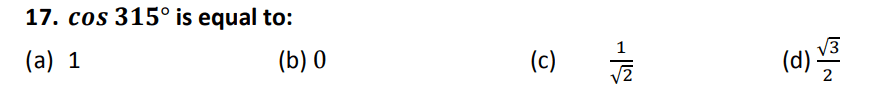81 / 100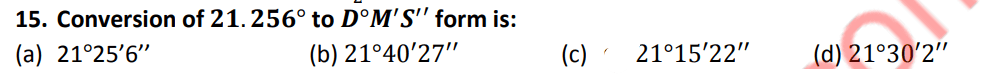82 / 10083 / 100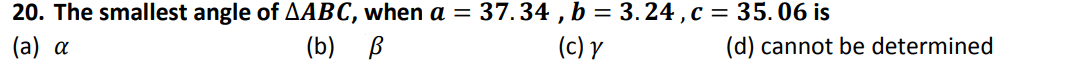84 / 100

The product of all four fourth roots of unity is:

85 / 100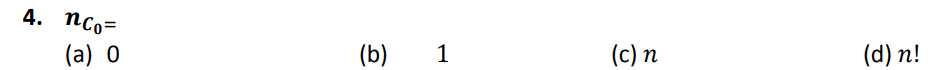86 / 10087 / 100

The chord containing the centre of the circle is

88 / 100

If all the sides of four sided polygon are equal but the four angles are not equal to 𝟗𝟎° each
then it is a

89 / 100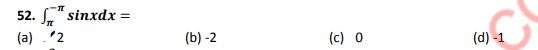90 / 100

Every homogeneous equation of 2nd degree in two variables represents

91 / 10092 / 10093 / 100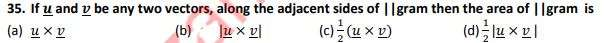94 / 100

Conditional equation 𝟐𝒙 + 𝟑 = 𝟎 holds when 𝒙 is equal to:

95 / 100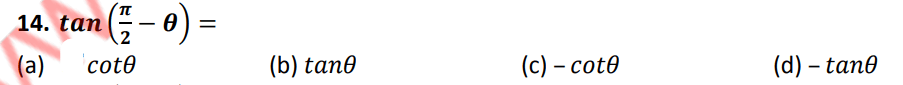96 / 100

If 𝒙 and 𝒚 have opposite signs then the point 𝑷(𝒙, 𝒚) lies the quadrants

97 / 100

The complex cube roots of unity are………………. each other.

98 / 100

The point where the medians of a triangle intersect is called_________ of the triangle.

99 / 100

Period of 𝒕𝒂𝒏𝟒𝒙 is

100 / 100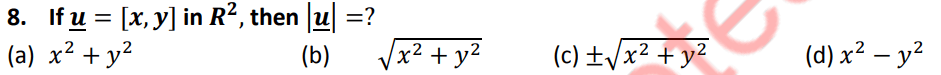The average score is 50%

0%

### One response to “FSC Math Full Book MCQs 2”

1. Anonymous says:

Why there only question marks in the place where no.s should be. Completely undoable and useless because of that.